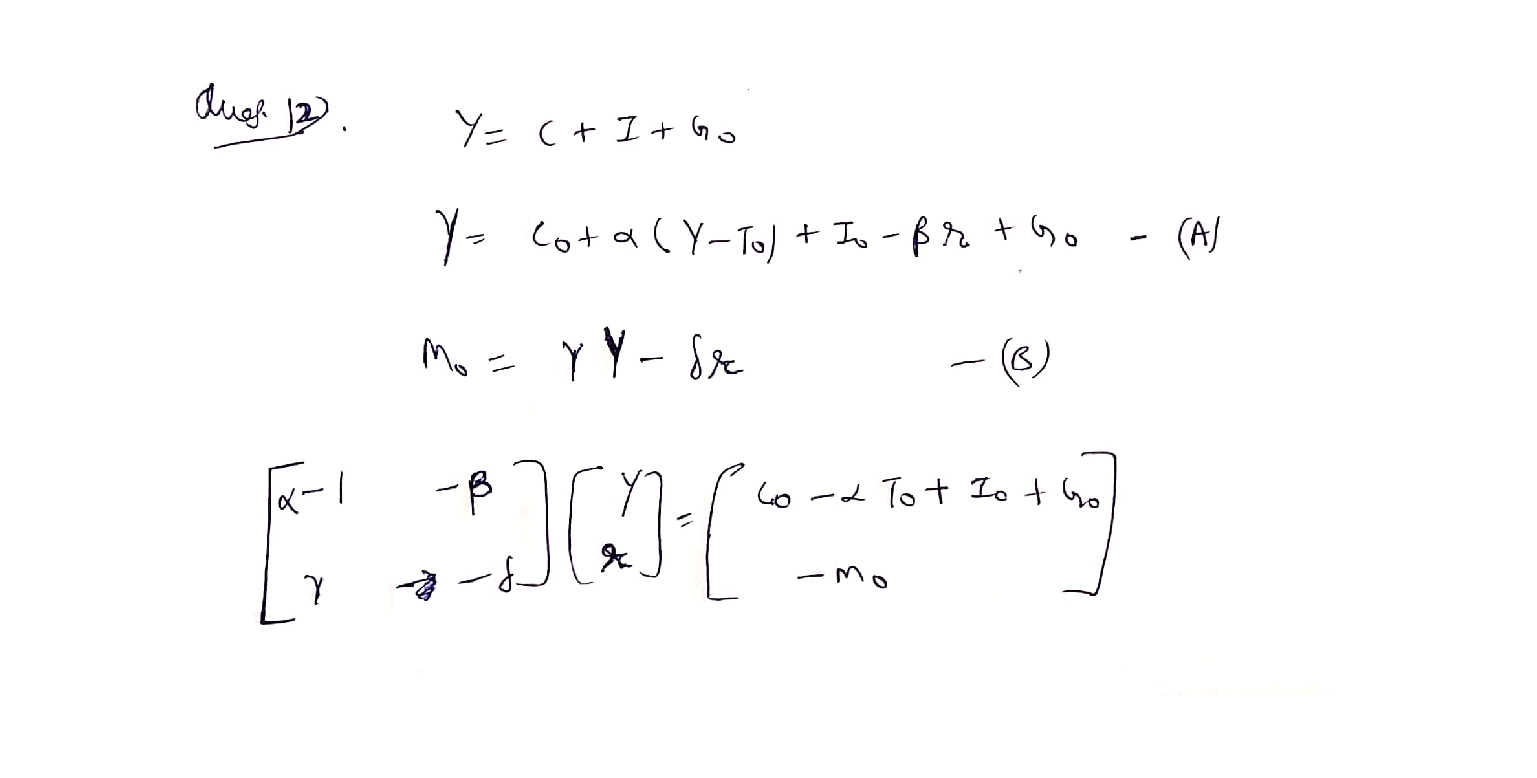Question

Linear Algebra

12. Consider the following linear IS/LM model in which Co, lo, Go, To, and Mo are exogenously determined and non-negative:

\begin{aligned} &Y=C+I+G_{0}\\ &C=C_{0}+a\left(Y-T_{0}\right) ; 0<a<1\\ &I=I_{0}-\beta r ; \beta>0\\ &-M_{0}=M^{d}\\ &M^{d}=\gamma Y-\delta r ; \quad \gamma, \delta>0 \end{aligned}

a. Substitute equations 2 and 3 into equation 1 and equation 4 into equation 5 to rewrite this model as a system of two linear equations in two unknowns: Y and r.

b. Write down the matrix equation (Ax = b) whose solution vector (x) describes the equilibrium combination of Y and r when x is defined as x =[Y* T*]

c. Use Cramer's rule to find the function that relates Y* to its exogenous determinants.

\text { d. Derive } \partial Y^{*} / \partial T_{0} \text { and } \partial Y^{*} / \partial M_{0}, \text { and determine their signs. }Verified### Question 52404Linear Algebra

In an election campaign, the popularity, B, as a percent of voters, of the governing Blue party can be modelled by a function of time, t, in days throughout the campaign as B(t) = 40 - 0.5t. The popularity, R(t), of the opposing Red party can be modelled by a composite function of B(t),R(B(t)) = 20 + 0.75[40 – B(t)].
Graph B(t) and describe the trend.

### Question 45665Linear Algebra

\text { 4] Find } g(0)-g(9)+g(2) \text {, if }
g(x)=\left\{\begin{aligned} \frac{x+1}{2}, & \text { if } x \text { is odd } \\ \frac{x}{2}, & \text { if } x \text { is even } \end{aligned}\right.

### Question 45664Linear Algebra

\text { 3] Find } f(4)-f(2)+f(3) \text {, if }
f(x)=\left\{\begin{array}{ll} \frac{x+1}{2}, & \text { if } x \text { is odd } \\ \frac{x}{4}, & \text { if } x \text { is even } \end{array}\right.

### Question 45663Linear Algebra

2] Find the Domains of the following functions:
\text { a) } f(x)=\sqrt{15-5 x}
f(x)=\frac{x^{2}-2 x+1}{x^{2}-4 x-21}
f(x)=\frac{x^{2}-2 x+1}{\sqrt{16-2 x}}

### Question 45662Linear Algebra

a) Give the definition of a rational function. [5 pts]
b) Give an example of a polynomial function of degree 3. [5 pts]
c) Can a constant function be a polynomial and a rational function at the same time? Explain your answer. [5 pts]
d) Give an example of a non-polynomial function and explain why not apolynomial function. [10 pts)

### Question 45333Linear Algebra

5) Ве,
\begin{array}{c} f: \mathbb{R} \rightarrow \mathbb{R} \\ f(x)=\left\{\begin{array}{ll} x^{2}-3 \cos (\pi x) & x<0 \\ x-4 e^{-2 x} & x \geq 0 . \end{array}\right. \end{array}
Calculate
\int_{-1}^{2} f(x) d x
Presenting the result in simplified form

### Question 45332Linear Algebra

4) Be f: R - Ra function differentiable in R such your derivative f', has in maximum a real zero.
Prove that the equation f(x)=0 has in maximum 2 real square

### Question 45331Linear Algebra

3) Take in consideration the following function
f(x)=\left\{\begin{array}{ll} f: \mathbb{R} \rightarrow \mathbb{R} & \\ \frac{x^{2}-4 x+\cos (\sin (x)),}{x^{4}+4 x^{2}+1}, & x \leq 0 \end{array}\right.
a) Show that the function f is continuous in R+ and in R- but discontinuous in the point X
b) Say justifying, if f is differenciable in X=0

### Question 45330Linear Algebra

2) Prove by definition that
\lim _{x \rightarrow 0} x^{2} \cos \left(e^{x}\right)=0

### Question 45329Linear Algebra

1) Calculate the following limit:
\lim _{x \rightarrow+\infty} \frac{x^{2}\left(e^{-3 x}+1\right)+x \cos (5 x)}{x^{2}+7 x+1}

### Submit query